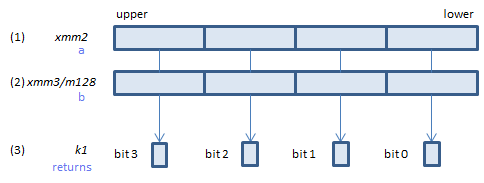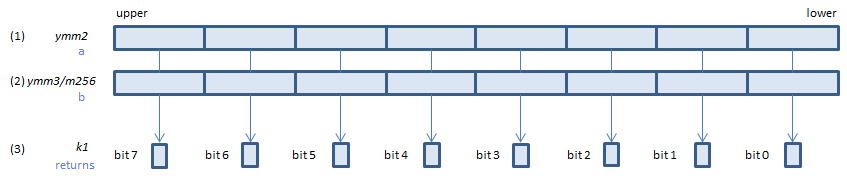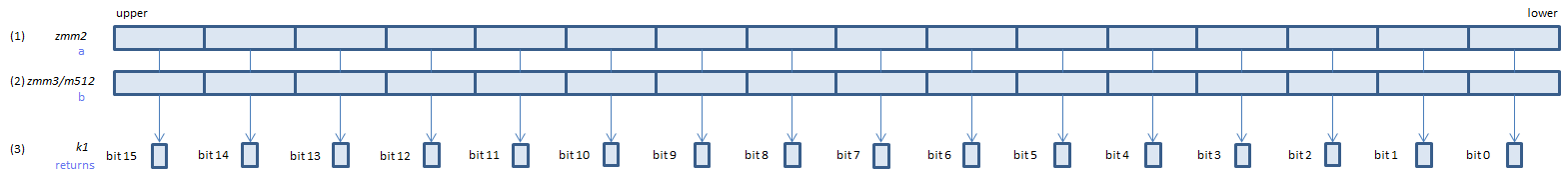﻿ vptestmd

## VPTESTMD - Packed TEST Mask Dword

VPTESTMD k1{k2}, xmm2, xmm3/m128/m32bcst    (V5+VLFor each DWORD, calculate bitwise (1) & (2), if any bit of the result is 1, set 1, else set 0, to the corresponding bit of (3).
If k2 bit is 0, the calculation is not done and the corresponding bit of (3) is set to zero. Upper bits of (3) are zero cleared.
VPTESTMD k1{k2}, ymm2, ymm3/m256/m32bcst    (V5+VL